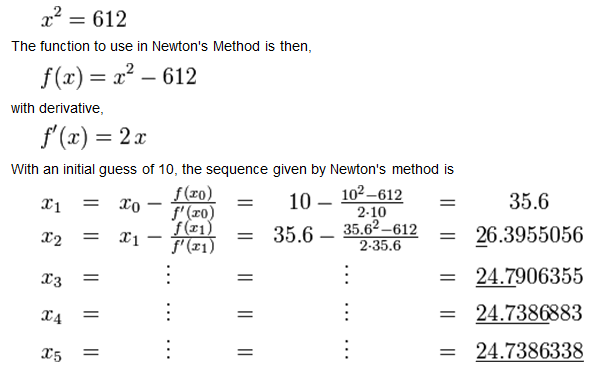# Happy Newton’s Day, 2008!So here we are, celebrating our 5th Newton's Day at 3QD. Along with Richard Dawkins, we independently and simultaneously came upon the idea of celebrating Newton's Day on December 25th in 2004, and each year since then I have written a little something about Sir Isaac. Here are my posts from 2004, 2005, 2006, and 2007. Today, I'll explain a simple but very useful mathematical technique called Newton's Method, discovered by Newton in 1669, though he published it later.

Newton's Method is a way of iteratively finding closer and closer solutions to a certain kind of mathematical equation (a real-valued function f(x), to be technical). We do this in the following way (it may help to look at the concrete example from Wikipedia in the graphic following my description of the general method, to better understand what I mean):

1. Take a guess at the solution (a value for x).
2. Plug in this value for x and see what f(x) is.
3. Draw a line tangent to the function at this point.
4. The point where this line intersects the x-axis is your new and improved value for x.
5. Take this new value for x and go back to step 2. (This repeating is called iteration.)Have a look at the graph (from Wikipedia) on the right. The function f(x) is the curved line shown in blue. In other words, for any point x on the horizontal axis, the blue line shows the value of f(x) on the vertical axis. The root (or solution) of the function is where the function crosses the horizontal axis; in other words, where the value of f(x) is zero. In the graph here, this is the value marked X. So we take a guess, Xn, go up the dotted blue line to the function, and then calculate the tangent line to the function at that point. This is shown in red. We see where that line crosses the x-axis, and take that point, Xn+1, as our value for x in the next iteration. As you can see, Xn+1 is a better approximation of the actual root, X. When we repeat the process over and over, one can very quickly converge on the correct solution.

How exactly do we do this? Let me show you. We know from calculus (as did Newton, since he invented it) that the derivative of a function f(x) at given point, written f'(xn), is just the slope of the tangent line at that point. But we also know that the slope of a line is just the “rise”, in this case, the value of f(xn) (on the vertical axis), divided by the “run,” the amount we move from Xn+1 to Xn on the horizontal axis. Setting these two quantities as equal, we get this equation: the derivative of the function at Xn is equal to the value of the function at Xn divided by the distance between Xn and Xn+1. In proper algebraic notation:

f'(xn) = f(xn) / (Xn – Xn+1)

Mutiplying both sides by (Xn – Xn+1), we get:

(Xn – Xn+1) * f'(xn) = f(xn)

Dividing both sides by f'(xn), we get:

(Xn – Xn+1) = f(xn) / f'(xn)

Subtracting Xn from both sides, we get:

– Xn+1 = f(xn) / f'(xn) – Xn

And finally, multiplying both sides by -1, we get:

Xn+1 = Xn – f(xn) / f'(xn)

So that's how once we make an initial guess Xn, we get our next value Xn+1. And then repeat the process.

Look at this nice example from Wikipedia, where one wants to find the square root of 612:In each successive result on the right hand side above, the correct digits are underlined. As you can see, one converges to a very accurate (to nine significant digits) approximation rather quickly. Well, there you go. That's Newton's Method. Interestingly, since computers are very fast at doing this sort of iteration, Newton's Method has become even much more useful now than when he invented it.

Oh, and if you don't understand “derivatives”, just take my word for it: the derivative of the function “x2 – 612” is “2x” and don't worry about the details… 🙂

I wish all of you a very happy holiday season, and also best wishes for a wonderful new year!